Using Small Script for a More Efficient Scanning Electron Microscope (SEM)

Scripts are short sections of coded software that make running specific experiments on a scanning electron microscope (SEM) more efficient.

This article is going to explore how the Phenom Programming Interface (PPI) can be used for image evaluation and centering.

Taking an SEM image can be considered to take place over four steps (Figure 1), which are:

• Image acquisition –Images are taken following the PPI using the Phenom SEM
• Analysis – Physical properties are determined using the image data
• Evaluation – The image properties are evaluated
• Reporting – The results of the process are made available to the user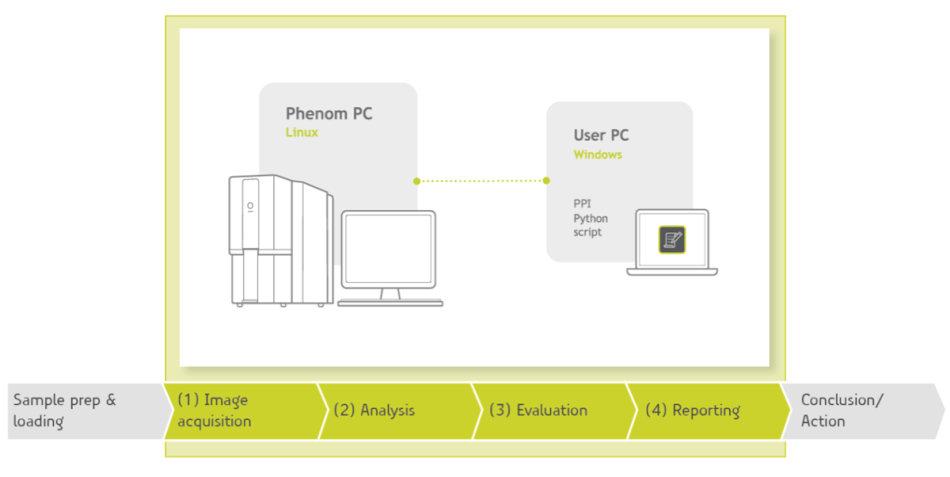Figure 1: Scanning Electron Microscopy workflow.

Image Evaluation

Image evaluation involves evaluating the physical properties extracted from the image (from the analysis step) and categorizing them. This is achieved by:

• Evaluating the extent of sample coverage the image provides
• Counting particle numbers and sorting according to their morphology
• Using the physical properties to center measurement on an area of interest (base action)

In article will base action on the physical properties in an image to determine where the center of the copper aluminum stub is.

In order to achieve this it must be assumed that the copper insert has a perfectly round shape. The script is then set up to begin searching from the location pstart, which is within the sample’s copper region.

From pstart searching takes place through the entire (positive and negative) x and y axes to locate four edge points of the copper region – Pleft, Pright, Ptop, Pbottom.

Assuming the perfectly circular structure of the copper region the center point, Pcenter, can be determined by taking the average of the x coordinates for (Pleft, Pright) and the y coordinates for (Ptop, Pbottom). Figure 2 illustrates this method.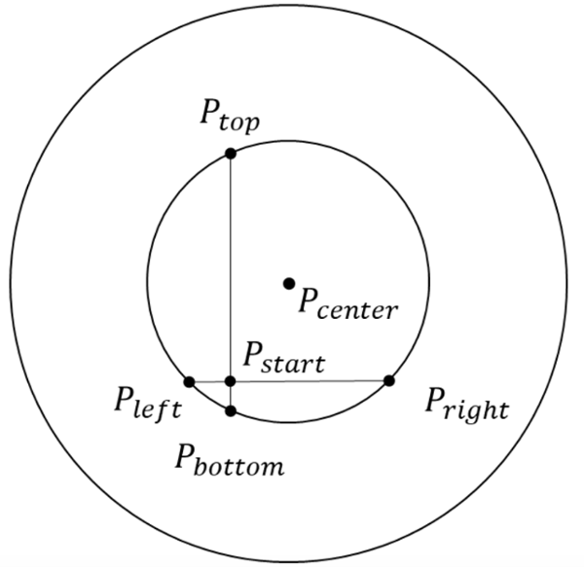Figure 2: Definitions of the locations on the stub.

The stage can be moved to find the edges, and at every step movement the image is segmented into material regions. The edge point can be recognized as the point at which less than 50% of the image contains a copper region, with the exact edge point defined as the center of the region that is not classified as either copper or aluminum.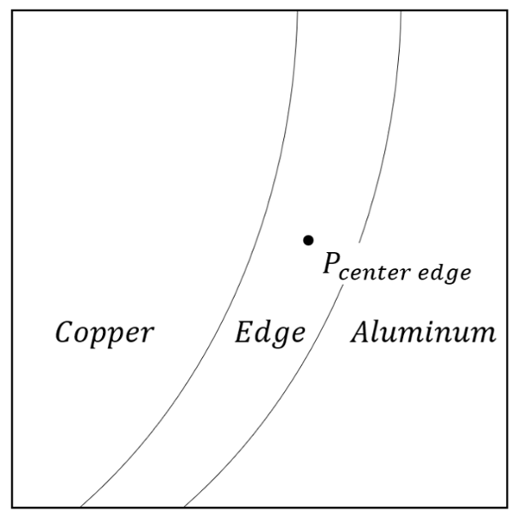Figure 3: Definitions of the locations on the stub.

An example of the script used to achieve this is shown in code snippet 1. Firstly Phenom.MoveTo is used to center the stage at its initial starting point, this position can be determined using the command phenom.GetStageModeAndPosition.

Following centering, the step size between images must be defined – half the image field width is an appropriate step size, which in this case is equal to 250 µm. Four vectors are defined in all directions to find the four edges. These vectors are combined into an iterable list, to be able to iterate over them in the for loop.

In the for loop, the stage is first moved to an initial guess of the location of the center, following which the stage is then moved step-wise and images are captured. The captured images are segmented and analyzed to determine if the copper region occupies less than 50% of the image area.

The edge has been found when the point at which copper occupies less than 50% of the image is reached, and the edge center (the ‘center of mass’) can then be determined using the function ndimage.measurements.center_of_mass.

The edge center is given in pixels, which can be converted into S.I. units using Phenom acquisition object’s metadata. The different edge centers are stored and once all of the data has been collected the locations (Pleft, Pright, Ptop, Pbottom) can be determined. The averages of these values are then taken to move the stage to the center location - Pcenter.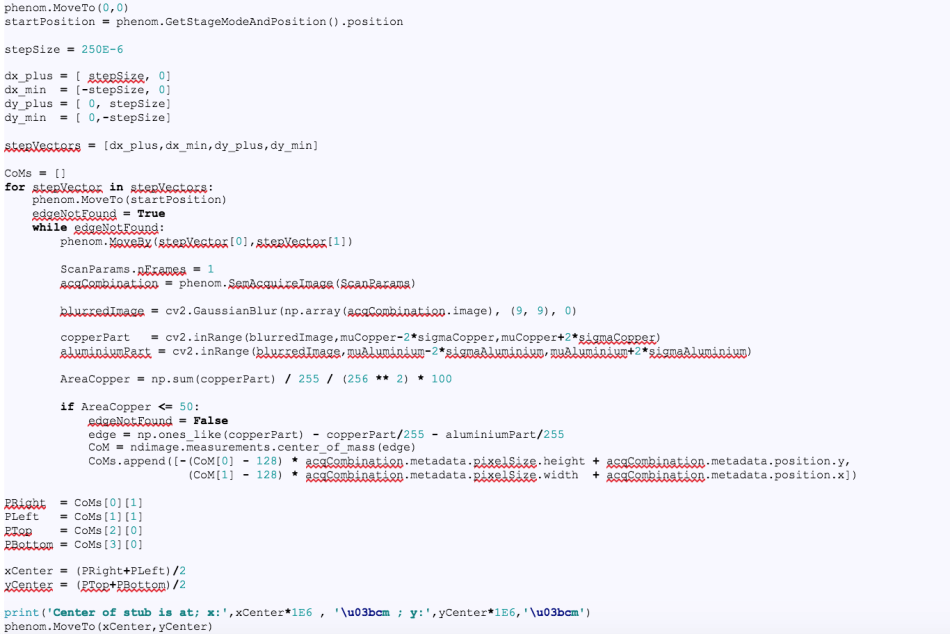Code snippet 1: Code to find and to move to the center of the stub.

This method provides a more accurate way of centering an SEM sample. Figure 4 shows the difference between the initial ‘center’ as inputted by the user (left) and the calculated center using the above method (right). Running the process multiple times can result in even more accurate centering, as improvements in symmetry mean the calculation’s assumptions are more valid.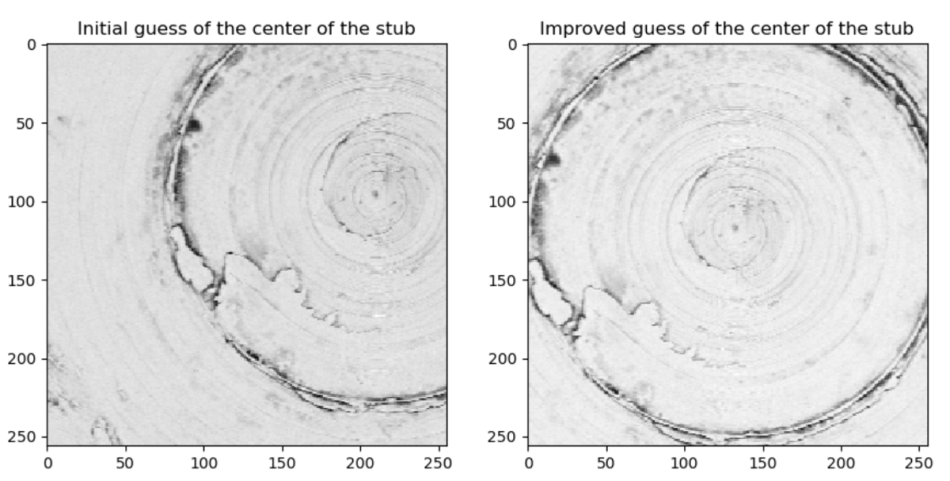Figure 4: Definitions of the locations on the stub.

The code for this entire procedure, including code for the acquisition and analysis stages, is shown in code snippet 2.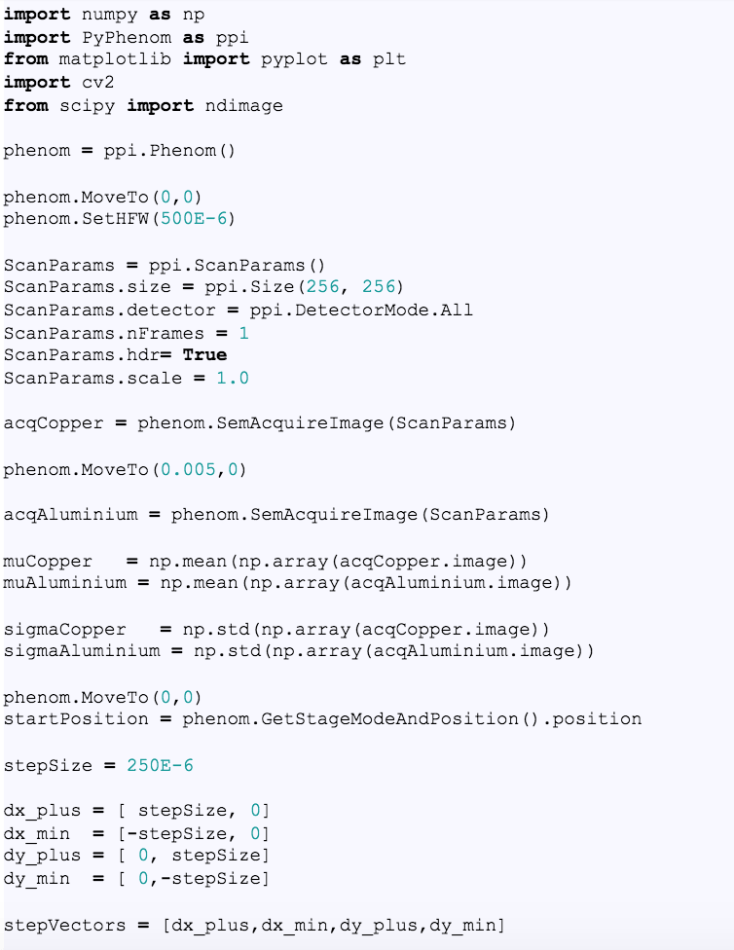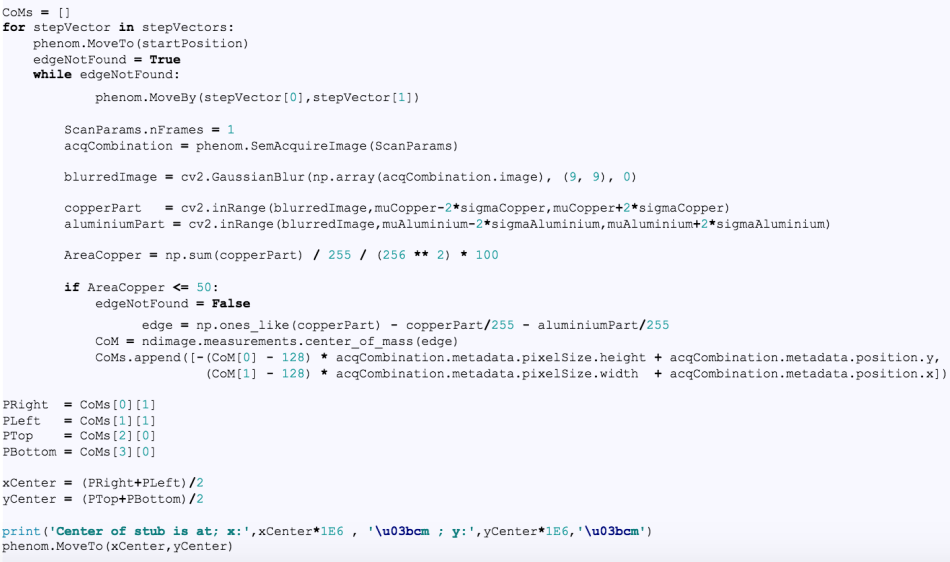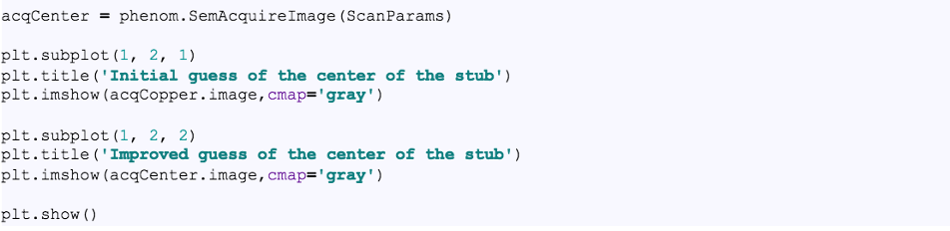Code snippet 2: Complete code.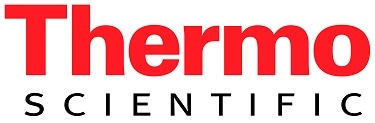This information has been sourced, reviewed and adapted from materials provided by Phenom-World BV.

For more information on this source, please visit Phenom-World BV.

Citations

Please use one of the following formats to cite this article in your essay, paper or report:

• APA

Thermo Fisher Scientific Phenom-World BV. (2018, November 20). Using Small Script for a More Efficient Scanning Electron Microscope (SEM). AZoM. Retrieved on October 23, 2019 from https://www.azom.com/article.aspx?ArticleID=17010.

• MLA

Thermo Fisher Scientific Phenom-World BV. "Using Small Script for a More Efficient Scanning Electron Microscope (SEM)". AZoM. 23 October 2019. <https://www.azom.com/article.aspx?ArticleID=17010>.

• Chicago

Thermo Fisher Scientific Phenom-World BV. "Using Small Script for a More Efficient Scanning Electron Microscope (SEM)". AZoM. https://www.azom.com/article.aspx?ArticleID=17010. (accessed October 23, 2019).

• Harvard

Thermo Fisher Scientific Phenom-World BV. 2018. Using Small Script for a More Efficient Scanning Electron Microscope (SEM). AZoM, viewed 23 October 2019, https://www.azom.com/article.aspx?ArticleID=17010.

Tell Us What You Think

Do you have a review, update or anything you would like to add to this article?